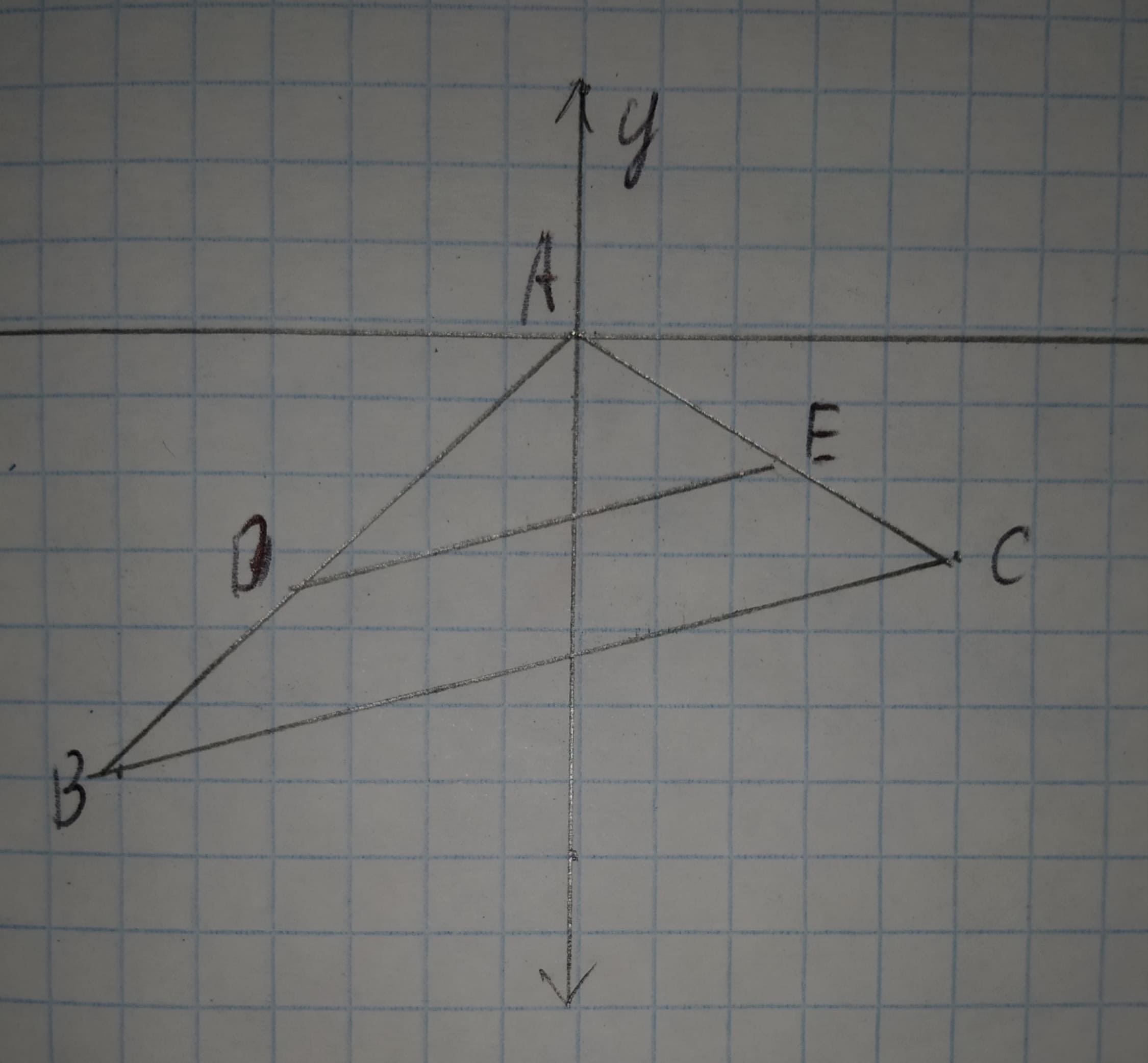# Check whether the given dilationis a similarity transformation or not.Given:The given dilation is12210202901.jpgpedzenekO 2020-10-27 Answered

Check whether the given dilationis a similarity transformation or not.
Given:
The given dilation isYou can still ask an expert for help

• Questions are typically answered in as fast as 30 minutes

Solve your problem for the price of one coffee

• Math expert for every subject
• Pay only if we can solve itMayme
Calculation:
From the figure, the given vertices are
A(0,0),B(-4,-4),C(4,-2),D(-2,-2),E(2,-1)
Find the distance (using distance formula) of corresponding sides and find the ratio.
$AB=\sqrt{{\left(-4-0\right)}^{2}+{\left(-4-0\right)}^{2}}=\sqrt{32}=4\sqrt{2}$
$AD=\sqrt{{\left(-2-0\right)}^{2}+{\left(-2-0\right)}^{2}}=\sqrt{8}=2\sqrt{2}$
$\frac{AD}{AB}=\frac{2\sqrt{2}}{4\sqrt{2}}=\frac{1}{2}$
$AC=\sqrt{{\left(4-0\right)}^{2}+{\left(-2-0\right)}^{2}}=\sqrt{20}=2\sqrt{5}$
$AE=\sqrt{{\left(2-0\right)}^{2}+{\left(-1-0\right)}^{2}}=\sqrt{5}$
$\frac{AE}{AC}=\frac{\sqrt{5}}{2\sqrt{5}}=\frac{1}{2}$
$BC=\sqrt{{\left[4-\left(-4\right)\right]}^{2}+{\left[-2-\left(-4\right)\right]}^{2}}=\sqrt{68}=2\sqrt{17}$
$DE=\sqrt{{\left[2-\left(-2\right)\right]}^{2}+{\left[-1-\left(-2\right)\right]}^{2}}=\sqrt{17}$
$\frac{DE}{BC}=\frac{\sqrt{17}}{2\sqrt{17}}=\frac{1}{2}$
From the above result, the lenght of the sides are proportional.
By the use of SSS similarity,
$\mathrm{△}ADE\sim \mathrm{△}ABC$.
Hence the given dilation is a similarity transformation.